Open in App
Not now

# ML | Bias Vs Variance

• Difficulty Level : Easy
• Last Updated : 20 Jul, 2021

In this article, we will learn ‘What are bias and variance for a machine learning model and what should be their optimal state.

There are various ways to evaluate a machine-learning model. We can use MSE (Mean Squared Error) for Regression; Precision, Recall and ROC (Receiver of Characteristics) for a Classification Problem along with Absolute Error. In a similar way, Bias and Variance help us in parameter tuning and deciding better-fitted models among several built.

Bias is one type of error that occurs due to wrong assumptions about data such as assuming data is linear when in reality, data follows a complex function. On the other hand, variance gets introduced with high sensitivity to variations in training data. This also is one type of error since we want to make our model robust against noise.

Before coming to the mathematical definitions, we need to know about random variables and functions. Let’s say, f(x) is the function which our given data follows. We will build few models which can be denoted as. Each point on this function is a random variable having the number of values equal to the number of models. To correctly approximate the true function f(x), we take expected value ofBias :Variance :Let’s see some visuals of what importance both of these terms hold.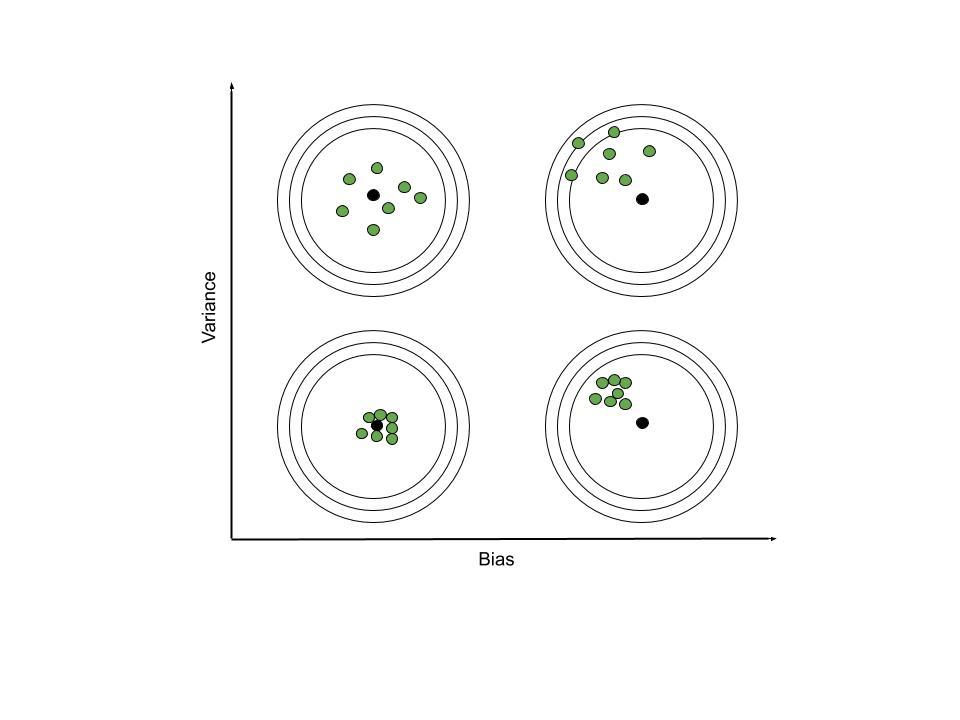These images are self-explanatory. Still, we’ll talk about the things to be noted. When bias is high, focal point of group of predicted function lie far from the true function. Whereas, when variance is high, functions from the group of predicted ones, differ much from one another.

Let’s take an example in the context of machine learning. The data taken here follows quadratic function of features(x) to predict target column(y_noisy). In real-life scenarios, data contains noisy information instead of correct values. Therefore, we have added 0 mean, 1 variance Gaussian Noise to the quadratic function values.xyy_noisy
-5252.67595670e+01
-4.520.252.11632561e+01
-4161.46802434e+01
-3.512.251.31647290e+01
-391.05460668e+01
-2.56.255.95794282e+00
-243.25487498e+00
-1.52.251.97478968e+00
-111.73960283e+00
-0.50.25-1.13112086e-02
001.64552536e+00
0.50.25-9.60938656e-01
114.46816845e-01
1.52.254.01016081e+00
241.54342469e+00
2.56.257.27654456e+00
399.37684917e+00
3.512.251.32076198e+01
4161.79133242e+01
4.520.252.08601281e+01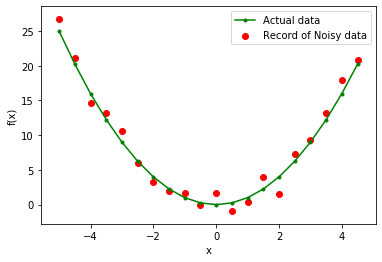Data Visualization

Now that we have a regression problem, let’s try fitting several polynomial models of different order. The results presented here are of degree: 1, 2, 10.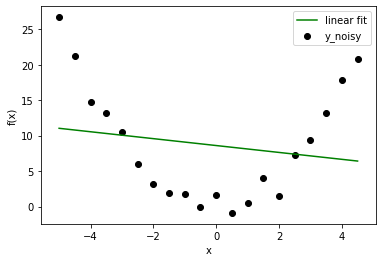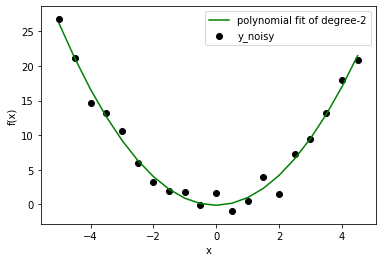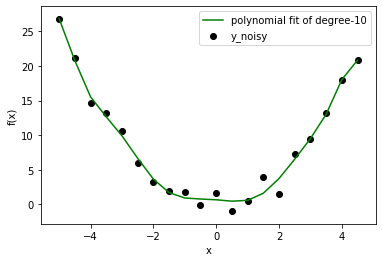In this case, we already know that the correct model is of degree=2. But as soon as you broaden your vision from a toy problem, you will face situations where you don’t know data distribution beforehand. So, if you choose a model with lower degree, you might not correctly fit data behavior (let data be far from linear fit). If you choose a higher degree, perhaps you are fitting noise instead of data. Lower degree model will anyway give you high error but higher degree model is still not correct with low error. So, what should we do? We can either use the Visualization method or we can look for better setting with Bias and Variance. ( Data scientists use only a portion of data to train the model and then use remaining to check the generalized behavior.)

Now, if we plot ensemble of models to calculate bias and variance for each polynomial model: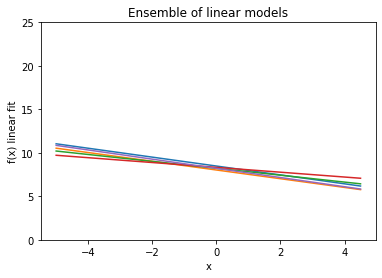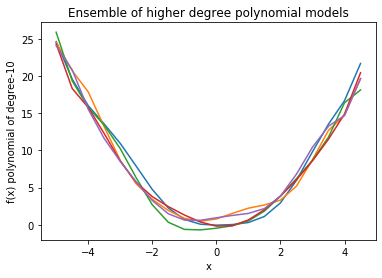As we can see, in linear model, every line is very close to one another but far away from actual data. On the other hand, higher degree polynomial curves follow data carefully but have high differences among them. Therefore, bias is high in linear and variance is high in higher degree polynomial. This fact reflects in calculated quantities as well.

Linear Model:-
Bias : 6.3981120643436356
Variance : 0.09606406047494431

Higher Degree Polynomial Model:-
Bias : 0.31310660249287225
Variance : 0.565414017195101


After this task, we can conclude that simple model tend to have high bias while complex model have high variance. We can determine under-fitting or over-fitting with these characteristics.

Again coming to the mathematical part: How are bias and variance related to the empirical error (MSE which is not true error due to added noise in data) between target value and predicted value.Now, let’s calculate another quantity:Now, we reach the conclusion phase. Important thing to remember is bias and variance have trade-off and in order to minimize error, we need to reduce both. This means that we want our model prediction to be close to the data (low bias) and ensure that predicted points don’t vary much w.r.t. changing noise (low variance).

My Personal Notes arrow_drop_up